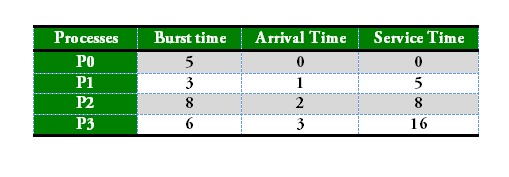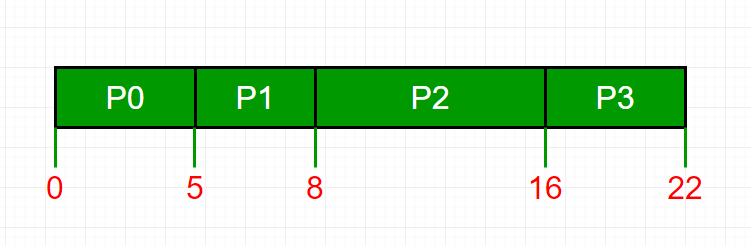Skip to content
Related Articles
Program for FCFS CPU Scheduling | Set 2 (Processes with different arrival times)
• Difficulty Level : Easy
• Last Updated : 01 Jun, 2021

We have already discussed FCFS Scheduling of processes with same arrival time. In this post, scenarios, when processes have different arrival times, are discussed. Given n processes with their burst times and arrival times, the task is to find average waiting time and an average turn around time using FCFS scheduling algorithm.
FIFO simply queues processes in the order they arrive in the ready queue. Here, the process that comes first will be executed first and next process will start only after the previous gets fully executed.

1. Completion Time: Time at which the process completes its execution.
2. Turn Around Time: Time Difference between completion time and arrival time. Turn Around Time = Completion Time – Arrival Time
3. Waiting Time(W.T): Time Difference between turn around time and burst time.
Waiting Time = Turn Around Time – Burst Time.```Process     Wait Time : Service Time - Arrival Time
P0                        0 - 0   = 0
P1                        5 - 1   = 4
P2                        8 - 2   = 6
P3                        16 - 3  = 13

Average Wait Time: (0 + 4 + 6 + 13) / 4 = 5.75```

Service Time: Service time means amount of time after which a process can start execution. It is summation of burst time of previous processes (Processes that came before)
Changes in code as compare to code of FCFS with same arrival time:
To find waiting time: Time taken by all processes before the current process to be started (i.e. burst time of all previous processes) – arrival time of current process
wait_time[i] = (bt + bt +…… bt[i-1] ) – arrival_time[i]
Implementation:

```1- Input the processes along with their burst time(bt)
and arrival time(at)
2- Find waiting time for all other processes i.e. for
a given process  i:
wt[i] = (bt + bt +...... bt[i-1]) - at[i]
3- Now find turn around time
= waiting_time + burst_time for all processes
4- Average waiting time =
total_waiting_time / no_of_processes
5- Average turn around time =
total_turn_around_time / no_of_processes```

## C++

 `// C++ program for implementation of FCFS``// scheduling with different arrival time``#include``using` `namespace` `std;` `// Function to find the waiting time for all``// processes``void` `findWaitingTime(``int` `processes[], ``int` `n, ``int` `bt[],``                                   ``int` `wt[], ``int` `at[])``{``    ``int` `service_time[n];``    ``service_time = at;``    ``wt = 0;` `    ``// calculating waiting time``    ``for` `(``int` `i = 1; i < n ; i++)``    ``{``        ``// Add burst time of previous processes``        ``service_time[i] = service_time[i-1] + bt[i-1];` `        ``// Find waiting time for current process =``        ``// sum - at[i]``        ``wt[i] = service_time[i] - at[i];` `        ``// If waiting time for a process is in negative``        ``// that means it is already in the ready queue``        ``// before CPU becomes idle so its waiting time is 0``        ``if` `(wt[i] < 0)``            ``wt[i] = 0;``    ``}``}` `// Function to calculate turn around time``void` `findTurnAroundTime(``int` `processes[], ``int` `n, ``int` `bt[],``                                      ``int` `wt[], ``int` `tat[])``{``    ``// Calculating turnaround time by adding bt[i] + wt[i]``    ``for` `(``int` `i = 0; i < n ; i++)``        ``tat[i] = bt[i] + wt[i];``}` `// Function to calculate average waiting and turn-around``// times.``void` `findavgTime(``int` `processes[], ``int` `n, ``int` `bt[], ``int` `at[])``{``    ``int` `wt[n], tat[n];` `    ``// Function to find waiting time of all processes``    ``findWaitingTime(processes, n, bt, wt, at);` `    ``// Function to find turn around time for all processes``    ``findTurnAroundTime(processes, n, bt, wt, tat);` `    ``// Display processes along with all details``    ``cout << ``"Processes "` `<< ``" Burst Time "` `<< ``" Arrival Time "``         ``<< ``" Waiting Time "` `<< ``" Turn-Around Time "``         ``<< ``" Completion Time \n"``;``    ``int` `total_wt = 0, total_tat = 0;``    ``for` `(``int` `i = 0 ; i < n ; i++)``    ``{``        ``total_wt = total_wt + wt[i];``        ``total_tat = total_tat + tat[i];``        ``int` `compl_time = tat[i] + at[i];``        ``cout << ``" "` `<< i+1 << ``"\t\t"` `<< bt[i] << ``"\t\t"``             ``<< at[i] << ``"\t\t"` `<< wt[i] << ``"\t\t "``             ``<< tat[i]  <<  ``"\t\t "` `<< compl_time << endl;``    ``}` `    ``cout << ``"Average waiting time = "``         ``<< (``float``)total_wt / (``float``)n;``    ``cout << ``"\nAverage turn around time = "``         ``<< (``float``)total_tat / (``float``)n;``}` `// Driver code``int` `main()``{``    ``// Process id's``    ``int` `processes[] = {1, 2, 3};``    ``int` `n = ``sizeof` `processes / ``sizeof` `processes;` `    ``// Burst time of all processes``    ``int` `burst_time[] = {5, 9, 6};` `    ``// Arrival time of all processes``    ``int` `arrival_time[] = {0, 3, 6};` `    ``findavgTime(processes, n, burst_time, arrival_time);` `    ``return` `0;``}`

## Java

 `// Java program for implementation of FCFS``// scheduling with different arrival time``public` `class` `GFG{`  `// Function to find the waiting time for all``// processes``static` `void` `findWaitingTime(``int` `processes[], ``int` `n, ``int` `bt[], ``int` `wt[], ``int` `at[])``{``    ``int` `service_time[] = ``new` `int``[n];``    ``service_time[``0``] = at[``0``];``    ``wt[``0``] = ``0``;` `    ``// calculating waiting time``    ``for` `(``int` `i = ``1``; i < n ; i++)``    ``{``        ``//representing wasted time in queue``        ``int` `wasted=``0``;``        ``// Add burst time of previous processes``        ``service_time[i] = service_time[i-``1``] + bt[i-``1``];` `        ``// Find waiting time for current process =``        ``// sum - at[i]``        ``wt[i] = service_time[i] - at[i];` `        ``// If waiting time for a process is in negative``        ``// that means it is already in the ready queue``        ``// before CPU becomes idle so its waiting time is 0``        ``// wasted time is basically time for process to wait after a process is over``        ``if` `(wt[i] < ``0``) {``            ``wasted = Math.abs(wt[i]);``            ``wt[i] = ``0``;``        ``}``        ``//Add wasted time``        ``service_time[i] = service_time[i] + wasted;``    ``}``}` `// Function to calculate turn around time``static` `void` `findTurnAroundTime(``int` `processes[], ``int` `n, ``int` `bt[],``                                    ``int` `wt[], ``int` `tat[])``{``    ``// Calculating turnaround time by adding bt[i] + wt[i]``    ``for` `(``int` `i = ``0``; i < n ; i++)``        ``tat[i] = bt[i] + wt[i];``}` `// Function to calculate average waiting and turn-around``// times.``static` `void` `findavgTime(``int` `processes[], ``int` `n, ``int` `bt[], ``int` `at[])``{``    ``int` `wt[] = ``new` `int``[n], tat[] = ``new` `int``[n];` `    ``// Function to find waiting time of all processes``    ``findWaitingTime(processes, n, bt, wt, at);` `    ``// Function to find turn around time for all processes``    ``findTurnAroundTime(processes, n, bt, wt, tat);` `    ``// Display processes along with all details``    ``System.out.print(``"Processes "` `+ ``" Burst Time "` `+ ``" Arrival Time "``        ``+ ``" Waiting Time "` `+ ``" Turn-Around Time "``        ``+ ``" Completion Time \n"``);``    ``int` `total_wt = ``0``, total_tat = ``0``;``    ``for` `(``int` `i = ``0` `; i < n ; i++)``    ``{``        ``total_wt = total_wt + wt[i];``        ``total_tat = total_tat + tat[i];``        ``int` `compl_time = tat[i] + at[i];``        ``System.out.println(i+``1` `+ ``"\t\t"` `+ bt[i] + ``"\t\t"``            ``+ at[i] + ``"\t\t"` `+ wt[i] + ``"\t\t "``            ``+ tat[i] + ``"\t\t "` `+ compl_time);``    ``}` `    ``System.out.print(``"Average waiting time = "``        ``+ (``float``)total_wt / (``float``)n);``    ``System.out.print(``"\nAverage turn around time = "``        ``+ (``float``)total_tat / (``float``)n);``}` `// Driver code` `    ``public` `static` `void` `main(String args[]) {``        ``// Process id's``    ``int` `processes[] = {``1``, ``2``, ``3``};``    ``int` `n = processes.length;` `    ``// Burst time of all processes``    ``int` `burst_time[] = {``5``, ``9``, ``6``};` `    ``// Arrival time of all processes``    ``int` `arrival_time[] = {``0``, ``3``, ``6``};` `    ``findavgTime(processes, n, burst_time, arrival_time);` `    ``}``}` `/*This code is contributed by PrinciRaj1992*/`

## Python3

 `# Python3 program for implementation of FCFS``# scheduling with different arrival time` `# Function to find the waiting time``# for all processes``def` `findWaitingTime(processes, n, bt, wt, at):``    ``service_time ``=` `[``0``] ``*` `n``    ``service_time[``0``] ``=` `0``    ``wt[``0``] ``=` `0` `    ``# calculating waiting time``    ``for` `i ``in` `range``(``1``, n):``        ` `        ``# Add burst time of previous processes``        ``service_time[i] ``=` `(service_time[i ``-` `1``] ``+``                                     ``bt[i ``-` `1``])` `        ``# Find waiting time for current``        ``# process = sum - at[i]``        ``wt[i] ``=` `service_time[i] ``-` `at[i]` `        ``# If waiting time for a process is in``        ``# negative that means it is already``        ``# in the ready queue before CPU becomes``        ``# idle so its waiting time is 0``        ``if` `(wt[i] < ``0``):``            ``wt[i] ``=` `0``    ` `# Function to calculate turn around time``def` `findTurnAroundTime(processes, n, bt, wt, tat):``    ` `    ``# Calculating turnaround time by``    ``# adding bt[i] + wt[i]``    ``for` `i ``in` `range``(n):``        ``tat[i] ``=` `bt[i] ``+` `wt[i]`  `# Function to calculate average waiting``# and turn-around times.``def` `findavgTime(processes, n, bt, at):``    ``wt ``=` `[``0``] ``*` `n``    ``tat ``=` `[``0``] ``*` `n` `    ``# Function to find waiting time``    ``# of all processes``    ``findWaitingTime(processes, n, bt, wt, at)` `    ``# Function to find turn around time for``    ``# all processes``    ``findTurnAroundTime(processes, n, bt, wt, tat)` `    ``# Display processes along with all details``    ``print``(``"Processes   Burst Time   Arrival Time     Waiting"``,``          ``"Time   Turn-Around Time  Completion Time \n"``)``    ``total_wt ``=` `0``    ``total_tat ``=` `0``    ``for` `i ``in` `range``(n):` `        ``total_wt ``=` `total_wt ``+` `wt[i]``        ``total_tat ``=` `total_tat ``+` `tat[i]``        ``compl_time ``=` `tat[i] ``+` `at[i]``        ``print``(``" "``, i ``+` `1``, ``"\t\t"``, bt[i], ``"\t\t"``, at[i],``              ``"\t\t"``, wt[i], ``"\t\t "``, tat[i], ``"\t\t "``, compl_time)` `    ``print``(``"Average waiting time = %.5f "``%``(total_wt ``/``n))``    ``print``(``"\nAverage turn around time = "``, total_tat ``/` `n)` `# Driver code``if` `__name__ ``=``=``"__main__"``:``    ` `    ``# Process id's``    ``processes ``=` `[``1``, ``2``, ``3``]``    ``n ``=` `3` `    ``# Burst time of all processes``    ``burst_time ``=` `[``5``, ``9``, ``6``]` `    ``# Arrival time of all processes``    ``arrival_time ``=` `[``0``, ``3``, ``6``]` `    ``findavgTime(processes, n, burst_time,``                            ``arrival_time)` `# This code is contributed``# Shubham Singh(SHUBHAMSINGH10)`

## C#

 `// C# program for implementation of FCFS``// scheduling with different arrival time``using` `System;``    ` `public` `class` `GFG{`` ` ` ` `// Function to find the waiting time for all``// processes``static` `void` `findWaitingTime(``int` `[]processes, ``int` `n, ``int` `[]bt, ``int` `[]wt, ``int` `[]at)``{``    ``int` `[]service_time = ``new` `int``[n];``    ``service_time = 0;``    ``wt = 0;`` ` `    ``// calculating waiting time``    ``for` `(``int` `i = 1; i < n ; i++)``    ``{``        ``// Add burst time of previous processes``        ``service_time[i] = service_time[i-1] + bt[i-1];`` ` `        ``// Find waiting time for current process =``        ``// sum - at[i]``        ``wt[i] = service_time[i] - at[i];`` ` `        ``// If waiting time for a process is in negative``        ``// that means it is already in the ready queue``        ``// before CPU becomes idle so its waiting time is 0``        ``if` `(wt[i] < 0)``            ``wt[i] = 0;``    ``}``}`` ` `// Function to calculate turn around time``static` `void` `findTurnAroundTime(``int` `[]processes, ``int` `n, ``int``[] bt,``                                    ``int` `[]wt, ``int``[] tat)``{``    ``// Calculating turnaround time by adding bt[i] + wt[i]``    ``for` `(``int` `i = 0; i < n ; i++)``        ``tat[i] = bt[i] + wt[i];``}`` ` `// Function to calculate average waiting and turn-around``// times.``static` `void` `findavgTime(``int` `[]processes, ``int` `n, ``int` `[]bt, ``int` `[]at)``{``    ``int` `[]wt = ``new` `int``[n]; ``int` `[]tat = ``new` `int``[n];`` ` `    ``// Function to find waiting time of all processes``    ``findWaitingTime(processes, n, bt, wt, at);`` ` `    ``// Function to find turn around time for all processes``    ``findTurnAroundTime(processes, n, bt, wt, tat);`` ` `    ``// Display processes along with all details``    ``Console.Write(``"Processes "` `+ ``" Burst Time "` `+ ``" Arrival Time "``        ``+ ``" Waiting Time "` `+ ``" Turn-Around Time "``        ``+ ``" Completion Time \n"``);``    ``int` `total_wt = 0, total_tat = 0;``    ``for` `(``int` `i = 0 ; i < n ; i++)``    ``{``        ``total_wt = total_wt + wt[i];``        ``total_tat = total_tat + tat[i];``        ``int` `compl_time = tat[i] + at[i];``        ``Console.WriteLine(i+1 + ``"\t\t"` `+ bt[i] + ``"\t\t"``            ``+ at[i] + ``"\t\t"` `+ wt[i] + ``"\t\t "``            ``+ tat[i] + ``"\t\t "` `+ compl_time);``    ``}`` ` `    ``Console.Write(``"Average waiting time = "``        ``+ (``float``)total_wt / (``float``)n);``    ``Console.Write(``"\nAverage turn around time = "``        ``+ (``float``)total_tat / (``float``)n);``}`` ` `// Driver code`` ` `    ``public` `static` `void` `Main(String []args) {``        ``// Process id's``    ``int` `[]processes = {1, 2, 3};``    ``int` `n = processes.Length;`` ` `    ``// Burst time of all processes``    ``int` `[]burst_time = {5, 9, 6};`` ` `    ``// Arrival time of all processes``    ``int` `[]arrival_time = {0, 3, 6};`` ` `    ``findavgTime(processes, n, burst_time, arrival_time);`` ` `    ``}``}``// This code is contributed by Princi Singh`

## Javascript

 ``

Output:

```Processes  Burst Time  Arrival Time  Waiting Time  Turn-Around Time  Completion Time
1        5        0        0         5         5
2        9        3        2         11         14
3        6        6        8         14         20
Average waiting time = 3.33333
Average turn around time = 10.0```

This article is contributed by Sahil Chhabra (akku). If you like GeeksforGeeks and would like to contribute, you can also write an article using write.geeksforgeeks.org or mail your article to contribute@geeksforgeeks.org. See your article appearing on the GeeksforGeeks main page and help other Geeks.
Please write comments if you find anything incorrect, or you want to share more information about the topic discussed above.

Attention reader! Don’t stop learning now. Get hold of all the important CS Theory concepts for SDE interviews with the CS Theory Course at a student-friendly price and become industry ready.

My Personal Notes arrow_drop_up